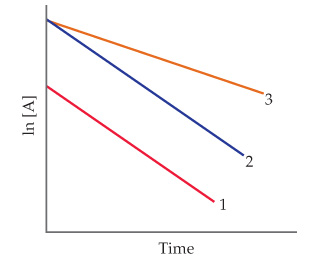# Problem: A friend studies a first-order reaction and obtains the following three graphs for experiments done at two different temperatures.Which two graphs represent experiments done at the same temperature? What accounts for the difference in these two graphs? In what way are they the same?

⚠️Our tutors found the solution shown to be helpful for the problem you're searching for. We don't have the exact solution yet.

###### Problem Details

A friend studies a first-order reaction and obtains the following three graphs for experiments done at two different temperatures.Which two graphs represent experiments done at the same temperature? What accounts for the difference in these two graphs? In what way are they the same?